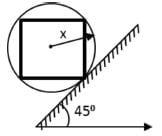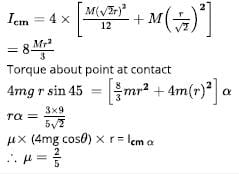For identical rods, each of mass m are welded...
For identical rods, each of mass m are welded at their ends to form a square, and the corners are then welded to a light metal hoop of radius r. If the rigid assembly of rods and hoop is allowed to roll down the inclined rough surface. If the minimum value of the coefficient of static friction which will prevent slipping is k/10
Find the value of k.• a)
a
• b)
b
• c)
c
• d)
d
Correct answer is '4'. Can you explain this answer?

### Related TestAbhishek Verma Sep 25, 2019Related For identical rods, each of mass m are welded at their ends to form a square, and the corners are then welded to a light metal hoop of radius r. If the rigid assembly of rods and hoop is allowed to roll down the inclined rough surface. If the minimum value of the coefficient of static friction which will prevent slipping isk/10Find the value of k.a)ab)bc)cd)dCorrect answer is '4'. Can you explain this answer?1 Crore+ students have signed up on EduRev. Have you?